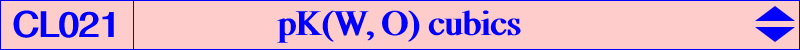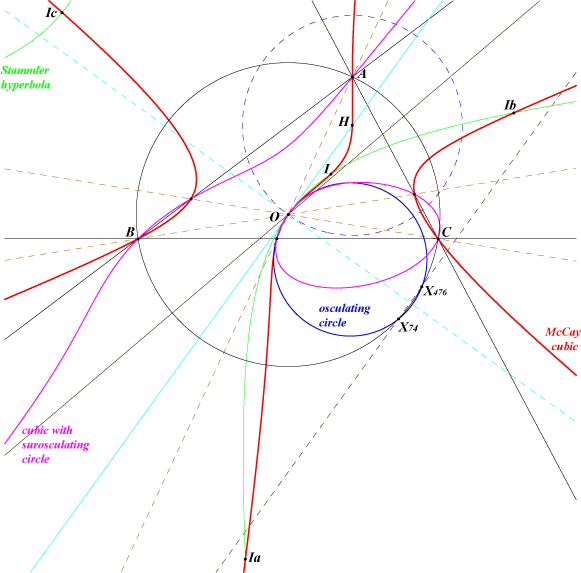Given an isoconjugation with pole W(p : q : r), the locus of point P such that the pedal triangles of P and its W-isoconjugate P* are orthologic is pK(W, X3), if we omit the line at infinity, its W-isoconjugate and the trilinear polar of the isotomic conjugate of the isogonal conjugate of W. See another generalization at K003 property 16. Compare this class of cubics with CL022. The barycentric equation of pK(W, O) is : ∑ a^2 SA x (r y^2 - q z^2) = 0.Special cases 1. Ki = K073 is the only cubic of this type which is circular. 2. The McCay cubic = K003 is the only cubic of this type which is a K60 and also which is isogonal. 3. K146 is the isotomic cubic of the class. 4. When W = X(577), the cubic decomposes into the cevian lines of X(3) since X(577) is the barycentric square of O. 5. The cubic is a pK+ if and only if W lies on Kw(O). See CL017. Kw(O) contains X(3) giving the McCay cubic, X(95), X(233) giving K413, X(577), the barycentric products X(3) x X(140), X(6) x X(3519). The following table gives a selection of pK(W, O) already mentioned in CTC.W cubic or X(i) on the cubic remarks X(6) K003 McCay cubic isogonal pK60+ X(50) K073 = Ki circular X(31) K145 X(2) K146 isotomic X(32) K172 X(2965) K349 X(233) K413 a pK++ X(14585) K576 X(257) K783pK(W, O) passing through a given point Q These cubics pK(W, O) form a net. Each cubic contains A, B, C, O and the vertices of the cevian triangle of O. Let Q be a triangle center distinct of O. pK(W, O) contains Q if and only if W lies on the line L(Q) through Q^2 (barycentric square) and O x Q (barycentic product). In this case, pK(W, O) also contains O/Q (cevian quotient) and all these cubics form a pencil since they have nine points in common. Note that L(Q) contains Q if and only if Q lies on the Euler line and, in this case, the cubic contains its pole W. Here is a selection of such cubics passing through some common triangle centers. Each cubic contains at least six centers among which O, Q and O/Q.Q O/Q W other centers X(i) on the cubic for i = remarks X(1) X(1745) X(41) 33, 55, 198, 259, 282 L(X1) is the line X(6)X(41). When W = X(6), we have the McCay cubic. X(56) 57, 278, 509 X(604) 34, 56, 266, 1035 X(1951) 21, 243, 851, 1936, 1937, 2656 X(2) X(3164) X(237) 6, 154, 232, 237, 511, 1297, 1976, 1987 L(X2) is the Euler line. pK(X2, X3) = K146 X(401) 95, 98, 401, 1972 X(4) X(1075) X(217) 6, 51, 154, 216, 418 L(X4) is the line X(4)X(6) X(2207) 19, 25, 1033 X(6) X(154) X(217) 4, 51, 216, 418, 1075 L(X6) is the line X(32)X(184) pK(X32, X3) = K172 X(237) 2, 232, 237, 511, 1297, 1976, 1987 X(1501) 31, 32, 1106, 1253, 1974 X(3051) 39, 66, 1843pK(W, O) with pole W on the Brocard axis X(577) is the barycentric square of O hence pK(X577, X3) degenerates into the cevian lines of O. Since X(577) lies on the Brocard axis, these cubics form a pencil such that – the tangent at O is the Euler line, – the polar conic of O is the Stammler hyperbola, – the osculating circle at O is independent of W. This is the circle through O, X(74) and X(476). This pencil contains several interesting cubics namely K003, K073, K172, K349. There is one and only one cubic such that the osculating circle becomes a surosculating circle but the pole is rather complicated.